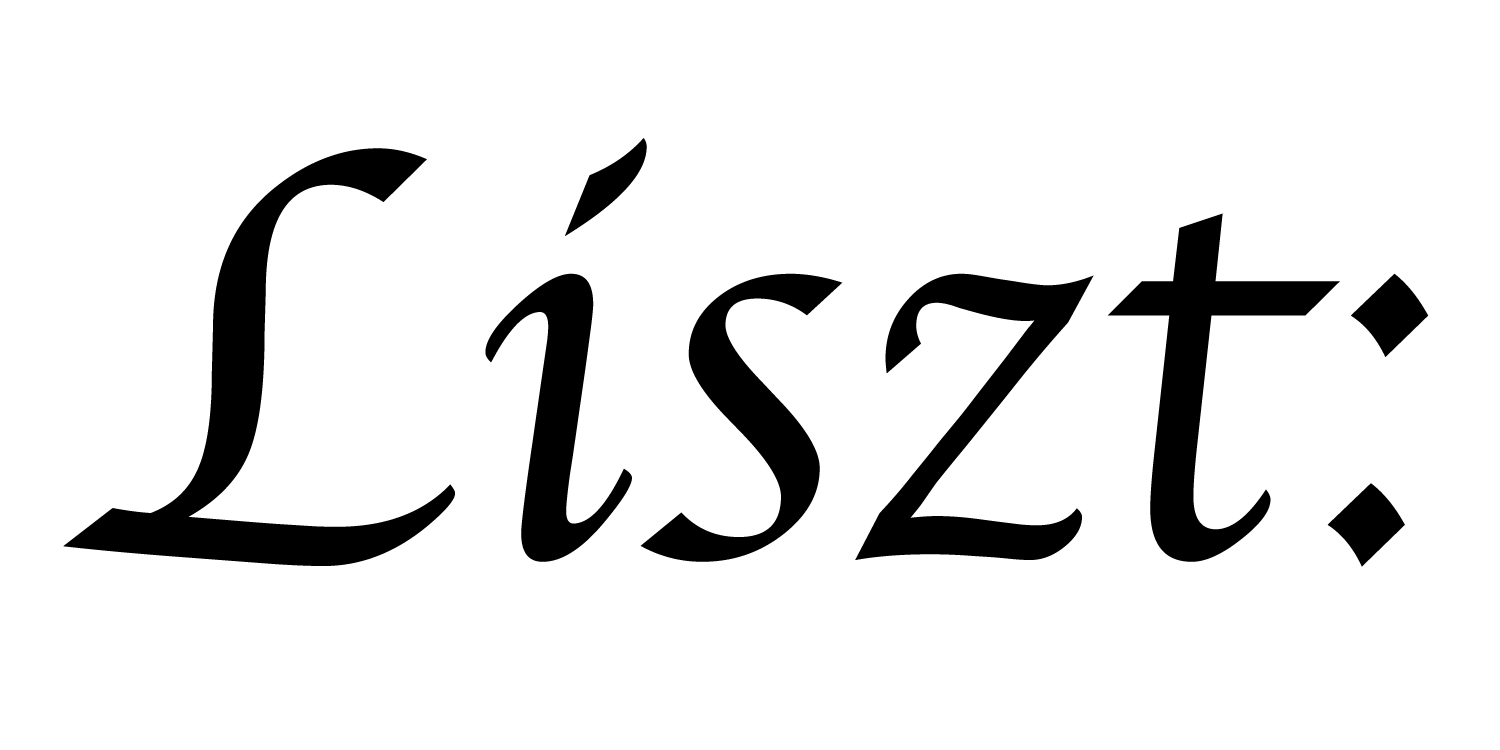# 07: Using Standard Grids

Part of what makes Ebb unique is the ability to handle simulations over very different domains. In the previous tutorials, other than the hello world, we used a triangle mesh domain. In this tutorial, we look at how to make use of the standard grid library.

``````import 'ebb'
local L = require 'ebblib'

local GridLib   = require 'ebb.domains.grid'

local vdb       = require 'ebb.lib.vdb'
``````

We start the program more or less identically, except we pull in the grid domain library instead of the OFF loader wrapper

``````local N = 40

local grid = GridLib.NewGrid2d {
size   = {N, N},
origin = {-N/2, -N/2},
width  = {N, N},
periodic_boundary = {true,false},
}
``````

Now, instead of loading the domain from a file, we can simply create the grid by specifying a set of named arguments. For a 2d grid, these are:

• size is the discrete dimensions of the grid; i.e. how many cells there are in the x and y directions. (here it is 40x40)
• origin is the spatial position of the (0,0) corner of the grid. (here we put the center of the grid at coordinate (0,0))
• width is the spatial dimensions of the grid (which we just put in 1-to-1 correspondence with the discrete dimension here)
• periodic_boundary is a list of flags indicating whether the given dimension should “wrap-around” or be treated as a hard boundary. (This argument is assumed to be {false,false} if not supplied) (Here, we set only the x direction to wrap around)
``````local timestep    = L.Constant(L.double, 0.45)
local conduction  = L.Constant(L.double, 1.0)
local max_diff    = L.Global(L.double, 0.0)

local function init_temperature(x_idx, y_idx)
if x_idx == 4 and y_idx == 6 then return 1000
else return 0 end
end
``````

Our definition of simulation quantities is more or less the same as for the triangle mesh. The one difference to remark on is that we define fields over `grid.cells` instead of `mesh.vertices`.

``````local ebb visualize ( c : grid.cells )
vdb.color({ 0.5 * c.t + 0.5, 0.5-c.t, 0.5-c.t })
var p2 = c.center
vdb.point({ p2, p2, 0 })
end
``````

Unsurprisingly, this means that the visualization code is also similar. However, because cells have spatial extent, it doesn’t make sense to simply look up their position. Instead, we ask for their `c.center` coordinates. (Warning: center is not actually a field, so if you try to write to it, e.g. `c.center = {3,4}` you’ll get an error)

``````local ebb update_temperature ( c : grid.cells )
var avg   = (1.0/4.0) * ( c(1,0).t + c(-1,0).t + c(0,1).t + c(0,-1).t )
var diff  = avg - c.t
c.new_t   = c.t + timestep * conduction * diff
end
``````

Our new temperature update step is similar to the double-buffer strategy from Tutorial 06, but we use the special grid mechanism for neighbor access. Here, we get the temperature from the four neighbors of a cell in the positive and negative x and y directions. In general, we can get the cell offset from the current one by (x,y) by writing `c(x,y)` and then access any fields on that cell.

``````local ebb measure_max_diff ( c : grid.cells )
var avg   = (1.0/4.0) * ( c(1,0).t + c(-1,0).t + c(0,1).t + c(0,-1).t )
var diff  = avg - c.t
max_diff  max= L.fabs(diff)
end
``````

The function to measure the maximum difference is essentially the same.

``````local ebb update_temp_boundaries ( c : grid.cells )
var avg : L.double
if c.yneg_depth > 0 then
avg = (1.0/4.0) * ( c(1,0).t + c(-1,0).t + c(0,1).t + c(0,0).t )
elseif c.ypos_depth > 0 then
avg = (1.0/4.0) * ( c(1,0).t + c(-1,0).t + c(0,0).t + c(0,-1).t )
end
var diff  = avg - c.t
c.new_t   = c.t + timestep * conduction * diff
end
``````

However, simply applying `update_temperature` is insufficient to handle the non-periodic boundaries in the y-direction. Instead, we’re going to need to somehow impose a boundary condition on our simulation.

By default, a non-periodic direction marks one final layer of cells on each side of the grid as part of the boundary. Then we can query how deep we are into a particular boundary using `c.yneg_depth` and `c.ypos_depth`. (Note that like `c.center` these “fields” can’t be assigned to)

``````for i=1,360 do
grid.cells.interior:foreach(update_temperature)
grid.cells.boundary:foreach(update_temp_boundaries)
grid.cells:Swap('t', 'new_t')

vdb.vbegin()
vdb.frame()
grid.cells:foreach(visualize)
vdb.vend()

if i % 10 == 0 then -- measure statistics every 10 steps
max_diff:set(0)
grid.cells.interior:foreach(measure_max_diff)
print( 'iteration #'..tostring(i), 'max gradient: ', max_diff:get() )
end
end
``````

Our simulation loop is mostly the same, but with one major difference. Rather than run `update_temperature` for each cell, we only run it for each `interior` cell. Likewise, we then execute the boundary computation only for each boundary cell. Though we still visualize all the cells with a single call. (Note that if we ran `update_temperature` on all of the cells, then we would produce array out of bound errors.)

a part of the Liszt project and PSAAP II center at Stanford University